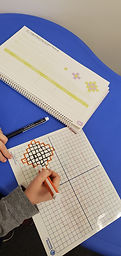Mr. Jake

Target 1​

Lesson Type:

Continuation

Critical Thinking

:

Logical Reasoning

Use reasoning to solve logic puzzles.

1:

Use the given information to determine which is not the correct answer.

2:

Use the given information in a problem to identify the correct answer.

3:

Follow given rules to solve problems.

4:

Think outside of the box to solve problems.

6th

Vocabulary:

Growing pattern

Activities:

Determined the rule for a growing patterns and used the rule to answer questions about the patternHome Exploration

Guiding Questions:Absent Students:

Target 2

:

1:

Identify the x- and y-axis on a coordinate plane.

2:

Locate points in all four quadrants of the coordinate plane.

3:

Recognize which quadrant a point is in, based on the properties of its coordinate pairs.

4:

Understand that the first number in a coordinate pair indicates placement on the x-axis and the second indicates placement on the y-axis.

5th

Vocabulary:

Coordinate, axis, point

Activities:

Plotted points in all 4 quadrants of the coordinate plane

Determined the coordinates of points on the coordinate planeHome Exploration

Guiding Questions:Target 3

:

Vocabulary:

Activities:Home Exploration